# Ultimate Guide To Graphing Calculators

As we progress through our education, we realize that math becomes more of a complicated subject. While we can solve equations by hand, you’ll end up wasting time of an exam. You need a high quality graphing calculator to save you time and answer exam questions more accurately.

This guide is to help you understand everything there is to know about graphing calculators. Before making a purchase, we suggest that you look in this guide not only to obtain a greater understanding, but to know what certain features you want in a calculator before you invest in it.

## Short History Of Graphing Calculators

The first commercially accepted and produced graphing calculator came from Casio. They created the calculator in 1984. It was able to graph mathematical functions and in later years, an icon menu was invented to help users navigate through the calculator a lot easier.In 1967, Texas Instruments invented the first handheld calculator. The calculator was small, sturdy, and easy to use. During 1990, they invented their first graphing calculator the TI-81. At the time, this calculator was popular amongst teachers and students.

In fact, the State of Conneticut required Texas Instruments to create 10,000 calculators for their students. After doing so, graphing calculators became the norm and we would see lesser scientific calculator models in production.

Chances are you still have an old graphing calculator found in your home. Graphing calculators make great family heirlooms because each manufacturer makes them to have a life expectancy up to 10 years. Because of this, graphing calculators are a valued tool for academic and professional mathematics.

## What Is A Graphing Calculator

A graphing calculator is needed in all forms of math. It’s a handheld computer that has the ability to plot graphs, performing tasks with variables, and solving simultaneous equations.

Most modern graphing calculators are programmable which helps the user create customized programs. Since they have large display screens, graphing calculators can show several lines of calculations and text at the same time.

Graphing calculators can perform the basic mathematical functions as normal calculators. All of the basic calculations and mathematical formulas are written on the calculator to find the correct answer.

The graphing calculator can perform functions such as the addition, division, subtraction, multiplication, square roots, decimals, fractions and a lot of other mathematical functions. When you type in the calculator the pre programmed functions and results will give you the answer.

## What Is The Computing System?

The computing system inside the graphing calculator is very complicated. Not only does it have to be programmed to solve geometry and algebraic equations, it must be programmed to draw out all of the solutions via a graph.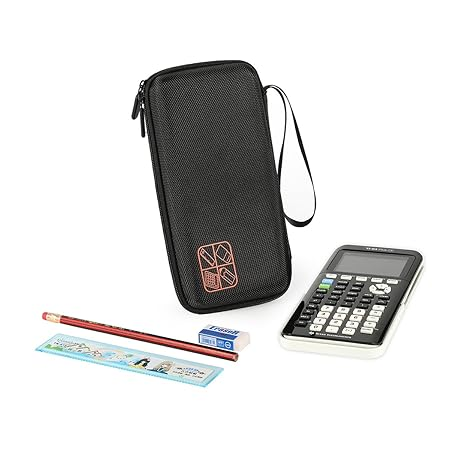The calculator gives formulas to carry out when the user begins typing in commands. Some common functions are plotting matrices, lines, and logarithms. If you type in “-10,10” the calculator will give out the correct solution.

## What Are The Graphing Capabilities?

At some times, the graphing capabilities of your calculator are highly limited. The results of your type equations (like -10,10) are then divided by how many pixels that are on the screen. For a 127 pixel screen, the graph will be divided by 127 dots that arcs across your calculator screen.

Sometimes the dots on the screen don’t fully connect. So you’ll have to manually reconnect them when you have to record them on a piece of paper. Unless you know where to place the graph coordinates, chances are you won’t find the correct answer. Graphing calculators input the literal commands you enter which might be completely off from the desired result.

## Graphing Calculator Vs. Scientific Calculator

Before the development of graphing calculators, scientific calculators were made. Scientific calculators are good at performing numerical computations and giving out the answers in a fraction or a decimal.

While scientific calculators are useful at performing basic math, you’re better off paying a for a graphing calculator. Graphing calculators are more expensive, but for a good reason. They allow you to see your progress on color coded graphs in a high resolution.

Graphing calculators take some time getting used to, but they give you a myriad of benefits. For instance, you can store multiple functions inside the calculator due to their enhanced memory and RAM. Once students pass the 9th grade, they should consider getting a graphing calculator.

## Programming Of A Graphing Calculator

Ever wanted your graphing calculator to handle long calculations without wasting time? That’s why you need to know how to program your calculator. Programming is the main feature behind graphing calculators and once you understand it, you’ll complete your math problems even faster.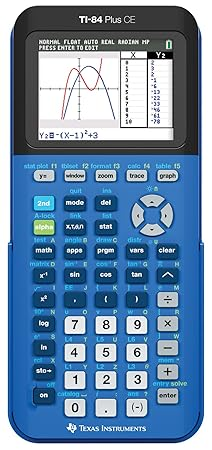For demonstration purposes, we’ll be programming the quadratic formula onto your calculator. Programs can be used to solve multiple math equations. Here is a list of some popular formulas you can program into your calculator:

• Reynold’s Number
• Pythagorean Theorem
• Antoine’s Equation
• Sphere Volume

• Get familiar with the calculator keys
• Press the PRGM button and give your program a new name
• Press ENTER, PRGM and then find the I/O Menu
• Show how you want the function to be displayed
• Press 2ND and ENTER on the equal sign
• Press PROMPT, and enter the new formula variables with the ALPHA key
• Type in the complete formula
• Go to PRGM, and scroll to the I/O Menu and then press DISP
• Tell the calculator your desired output
• Test out the program

## Reasons To Use A Graphing Calculator

Most graphing calculators, as well as some non-graphing scientific and programmer's calculators can be programmed to automate complex and frequently used series of calculations and those inaccessible from the keyboard.

### Algebra

Algebra is all about linear equations and finding the slope of certain x and y points. You can use a scientific calculator, but how do you physically see the results of your graphs? Use a graphing calculator because it’s easier to plug in the variables and plot your graph all in one neat screen.### Statistics

When it comes to statistics, you’ll need a calculator that can help you accurately display the meaning behind the numbers of a problem. With a graphing calculator you can find the mean, standard deviation, and compute complex statistical problems faster than you would by hand.

### Geometry

Geometry requires a lot of knowledge and understanding about angles and how they work together to make a shape. Get a graphing calculator so that you can plot triangles, circles, and find the diameter of odd shapes.

### Calculus

For college students, graphing calculators are mandatory. In questions where you have to find the derivative of a problem, a graphing calculator can do it within seconds. From Calculus I to Calculus III, the graphing calculator is a great tool to have on your side.

## Basic Features And Functions Of A Graphing Calculator

Graphing Calculator features a captivating color display that enables students to see equations, data and graphs clearly. Look at some other graph calc features:

### Graph Plotting

Plotting your graph on a graphing calculator is easy. First, find the x and y coordinates of the equation. After you do that, you press 2ND and GRAPH. Plug in the numbers into the X and Y into their respective places and there you go! You have a fully plotted graph!

### Finding Intersections

• Graph your functions in a viewing window that has the point of intersection of the function
• Use the [2ND][TRACE] buttons to activitate the Calculate menu
• Press  to find an intersecting option
• Select your first and section function
• Move the cursor right and left to show the cursor as close as it can be displayed evenly and perfectly
• Press [ENTER] to show the point of intersection coordinates

### Scientific Functions

Remember, graphing calculators have a huge advantage over scientific calculators. This is because they are able to not only perform scientific functions, but can display them in a way that’s easy for the student to see.

Here is a list of scientific functions that a graphing calculator can do:

• Sine, Cosine, Tangent (SIN, COS, TAN)
• Logarithmic Functions
• Order of Operations ()

Since a graphing calculator can do scientific functions, it’s better to save money and invest in a graphing calculator instead. You’ll have a visual and a scientific explanation behind each of your answers and you won’t have an issue solving complex mathematical problems.

Graphing calculators also have some additional features:

### Computer Algebra System

Computer Algebra System is a term used for any mathematical software that can manipulate multiple mathematical expressions in the same way a scientist of a mathematician perform their manual computations.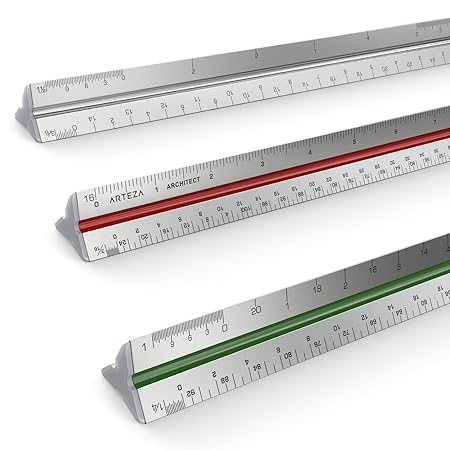The main goal of a computer algebra system is to automate difficult and sometimes tedious algebraic and calculus tasks. The largest difference between a computer algebra system and a non computer algebra system is that CAS calculators show their answers in symbolic and not numerical form.

The specific capabilities and uses of CAS calculators vary from one another. However, their purpose is still the same: to manipulate symbolic equations. CAS systems provide a programming language for users to define their own procedures and allows them to graph equations.

### Laboratory Usage

Most graphing calculators can be attached to a myriad of scientific devices. Some of those devices are:

• pH gauges
• Electronic thermometers
• Decibel and Light meters
• Accelerometers

Your graphing calculator can also be used on a school network and helps students interact with the teacher even faster. Having a graphing calculator enhances the student’s learning in math, especially in mechanics and statistics.

### Games

Graphing calculators provide a fun and entertaining way to learn math. Since most graphing calculators are user progammable, there is a large library of user created games that’s available.

It’s easy to download games onto your graphing calculator. Most graphing calculator brands are open source, which allows the users to make their own games with ease.

While handheld math gaming products are available, graphing calculators have superior games that improve the student’s knowledge in science and math.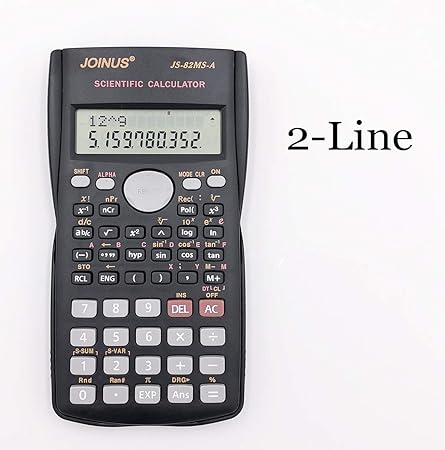But there is one disadvantage. Most graphing calculators with gaming software are banned by teachers, school institutions, and standardized test examinations. Make sure your graphing calculator is compatible with your school or exam to get the most out of it.

## What Is A CAS Calculator

CAS stands for Computer Algebraic System. Unlike scientific calculators, CAS calculators display the answers in a symbolic form. This is a great advantage for students as CAS calculators are able to complete math problems using the same notation as a mathematican.

Why do you need a CAS calculator? Because they make evaluating and completing equation problems less of a hassle. While some college students can benefit from using a CAS calculator, they are more suited for professionals who create symbolic expression often.

PROS

• SAT Advantages - Students who use a CAS calculator will save more time and will perform better on their SATS
• Intuitive - CAS calculators come with quick touch screen features that makes it easier to use. Get a CAS calculator because they work more like a PC instead of a complicated assembly language
• Powerful and Faster Speed - Instead of completing problems via paperwork, a CAS calculator is fast enough to perform mathematical computations in an instant

CONS

• Frequently Banned - Certain tests and teachers don’t allow CAS calculators during examination. For instance, the ACT bans CAS calculators because it would give students an unfair advantage
• Expensive - CAS has more advanced software than Non CAS models. This means that you’ll have to pay extra for a CAS calculator. However, you’ll be rewarded with the latest technology and will be able to complete problems faster than Non CAS calculators

## Graphing Calculator Benefits

A graphing calculator makes math classes, assignments, and exams a smoother and more automated experience. Here are a few benefits that you’ll notice as soon as you buy your first graphing calculator.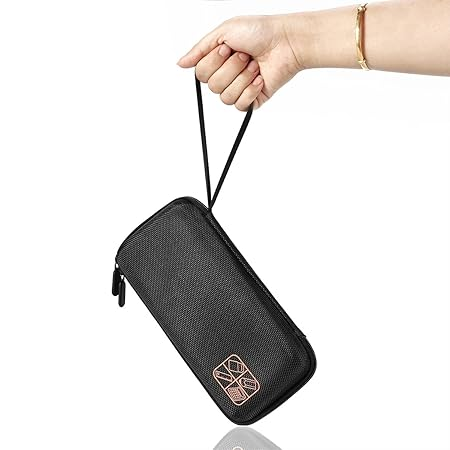### Accurate

Graphing calculators are accurate and fast, which makes them a great complementary device for students. For example, you can have your students complete the problem by hand and then use the calculator to check if their answer was correct.

Graphing calculators are great tools for this reason. If both of the answers aren’t the same, students can re-check their work to see where they might’ve made a mistake. Calculators are tools that allow students to validate their answers and should be used as help and not a crutch.

### Student Enjoyment

Math is one of the most complicated subjects in school, but using a graphing calculator helps students get the hang of it quicker. If you have a graphing calculator, you’ll be able to enjoy the class as it helps you get understand tough concepts easier.

Currently, we live in a society that always changes due to the innovative developments in technology. There are some classes that allow students to use Apple iPads for their work. Learning how to use this technology helps students become more confident and efficient.

Graphing calculators are simple tools to use in regards to electronics.They help students calculate taxes, set a budget, and complete other life related tasks. Students are going to use calculators throughout their lives so it’s important to learn how to use them now.

## Manufacturers

We compiled a list of our top graphing calculator manufacturers:

### Texas Instruments

Texas Instruments is an American technology company that’s known for selling calculators for all academic and professional levels. Headquarted and founded in Dallas, Texas, U.S. Texas Instruments focuses on creating analog chips and calculators for students and teachers.In 1990, Texas Instruments produced the TI-81 calculator. It became very popular amongst academic institutions and reinforces why they are a leader in the calculator industry. If you want a reliable calculator that’s backed by a high quality manufacturer, get a TI Calculator.

### Casio

Casio is a Japanese manufacturer that’s located in Tokyo, Japan. Their main products include digital cameras, mobile phones, digital watches, and calculators. Casio started in 1946 and created the first hand held calculator in 1957.

For users wanting a classic yet functional graphing calculator, look into Casio’s products. Each of them are designed in Casio’s signature black color and is compact enough to fit in your bookbag. Buy a Casio calculator now if you want a high end graphing calculator at an affordable price.

### HP

HP is a company who sells laptops, tablets, and graphing calculators. It’s known for having high quality graphing calculators that have new innovative features. For instance, every HP Calculator has a touchscreen display system; which is easier for intuitive students to use.

When buying from HP, you’ll also like its multiple apps. As soon as you turn the screen on, you’ll have 5 mathematical apps that are used to enhance your learning. Basically, HP is one of the best calculator manufacturers because of their new, and easy to use system.

## Note About Graphing Calculator On The SAT And AP Exams

Both exams have similar regulations when it comes to using graphing calculators on their exams. Before we start to speak on the different calculators they accept, you must understand the certain calculators and mathematical technology they’ve banned.

### Banned Calculators And Technology

Here is a list of the technology that students are prohibited from using on the SAT and AP:

• Calculators that can access the internet
• Calculators with a Bluetooth or wireless capacity
• Calculators with a scanning or camera feature
• Models with a video player/digital audio feature
• Calculators with a stylus/pen input
• Models with a QWERTY keyboard as part of their software or hardware

Fortunately, over 90% of the graphing calculators are able to be used during the exam. Check the above prohibited requirements to ensure that your calculator can be used on the exam. If your calculator is banned during the exam, the proctor will give you an extra one to use.

### AP Exam

For the AP exam, they’ve created a chart on which subjects are calculator friendly. Read the chart below to see if your calculator matches up with their standards.

### SAT Calculator Guidelines

In comparison to the AP exams, the SAT has a more lenient set of guidelines. Here are the rules regarding calculators in the SAT:

• Scientific Calculators of all models are accepted
• Some graphing calculators
• Four-function calculators

### SAT And AP Calculator Tips

If you don’t regularly use a calculator, try using it on a few practice problems before taking it to the exam. Use a calculator that you know and are familiar with to increase your final score.

You don’t have to use your calculator to answer every question. First, see if you can solve the problem. After that use the calculator to validate your answer. Your calculator is supposed to assist you during the exam and help with problem solving questions; not as a distraction.Make sure that you come with fully charged batteries and your calculator is in good working condition. Bring an extra pair of batteries in your backpack. If your calculator shuts off or malfunctions in the exam you can either cancel the test or work without the calculator.

Use scratch paper to get your thoughts down before entering information in a calculator.

## Conclusion

It doesn’t matter what your educational skill level is. A graphing calculator is useful for students, teachers, and professionals who want to take less time calculating math problems. Once you know how to fully use your graphing calculator, you’ll see a higher score on your exams.

But still, you need to know which calculator is right for you. We invite you to look into our buying guide where we show you the top 5 graphing calculators that are available. All of them are accepted in SAT, AP, and other major testing institutions, so you should read the guide and buy one today.